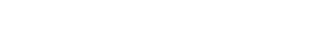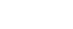## Mathematics Paper 2 Questions - Mangu High School Mock Exams 2022

### QUESTIONS

SECTION 1: 50 MARKS
Answer all questions in this section

1. Solve the following simultaneous equations. (4mks)
2x - y = 3
x2 - xy = -4
2. Calculate the standard deviation of the following set of numbers to two decimal places. (4mks)
10, 13, 14, 16, 17, 20
3. The base and perpendicular height of a triangle measured to the nearest cm are 6cm and 4cm respectively. Find the absolute error In calculating the area of the triangle. (2mks)
4. Solve for x in the following equation 4sinxcosx - sinx = 0 for -90° ≤ x ≤ 90° (4mks)
5. Simplify (3mks)
(√x + 2) (√x  - 1)
√x
6. A field is in the shape of an equilateral triangle ABC of sides 80m. The owner wishes to plant some flowers in the field. The flowers must be at most 60m from A and in the half of the field nearer to B than to C. If no flower is to be more than 40m from BC, using a scale of 1cm to represent 10m, show by shading the exact region where the flowers may be planted. (4mks)
7. Solve for x in the equation log5x + 1/log5x = 2  (3mks)
8. Find the equation of the normal to the curve y=x2 + 3x at the point where - 1 (3mks)
9. A belt is passing round two pulleys of radil 5cm and 10cm respectively making two exterior common tangents and two arcs. If the distance between the centers of the two pulleys ls 18cm, find the total length of the exterior common tangents to 1 decimal place. (2mks)
10. The points (2, 2) and (8,4) are the end points of a diameter of a circle. Write down the equation of the circle. (3mks)
11. Use the matrix method to solve the following simultaneous equations. (4mks)
2x + 2y = 1
x + 3y = 0
12. The variable y varies partly as x and partly as the Inverse of X. If y=13 when x=3 and y=12 when x=2, find the value of y when x = 6. (4mks)
13. The difference between the fourth and the seventh terms of an increasing arithmetic progression is 12. Calculate the sum of the first five terms of the progression if the first term is 9. (3mks)
14. Find the constant term in the expansion (2x + 1/x)6 (2mks)
15. Given that(3mks)
16. A boat leaves a port and salls 50km due east. It then changes direction and sails another 50km on a bearing of 200°. Calculate how far from the port the boat is. (3mks)
17.
1. Complete the following table for the equation y = x3 + 4x2 - x - 6 (2mks)
 x -5 -4 -3 -2 -1 0 1 2 x3 4x2 -x -6 y
2. Draw the graph of y = x3 + 4x2 - x - 6 for -5 ≤ x ≤ 2 (3mks)3. Using the graph, estimate the roots of the equation x3 + 4x2 - x - 6 = 0 (2mks)
4. Using the same graph, estimate the solution to the equation x3 + 4x2 - 12x = 0 (3mks)
18.
1. Use the mid-ordinate rule with 5 strips to estimate the area bounded by the curve y = x2 + x + 1, the y axis, x axis and the line 5. (3mks)
2. Estimate the same area using the trapezium rule with 6 ordinates. (3mks)
3. Calculate the exact area covered by the region (a) above. (2mks)
4. Find the percentage error in (a) and (b) above. (2mks)
19. The 1st, 7th and 25th terms of an arithmetic progression are the first three consecutive terms of a geometric progression. The 20 term of the arithmetic progression is 22. Find:
1.
1. The first term and common difference of the arithmetic progression. (4mks)
2. The sum of the first 40 terms of the arithmetic progression. (2mks)
2.
1. The 10th term of the geometric progression. (2mks)
2. The sum of the first 10 terms of the geometric progression. (2mks)
20. OABCD is a pyramid in which the horizontal base ABCD is a square of sides 8cm. If OA = OB= OC = OD=12cm.Find to four significant figures where applicable;
1. The vertical height of the pyramid (2mks)
2. The angle between planes OAB and ABCD (2mks)
3. The angle between planes OBC and OAD (2mks)
4. The angle between planes OAB and OBC (4mks)
21. The frequency distribution of marks of 110 students is given in the table below.
 Marks 1-10 11-20 21-30 31-40 41-50 51-60 61-70 71-80 81-90 91-100 Frequency 2 5 9 12 25 37 11 6 2 1
1. Draw an o-give curve to illustrate the data (4mks)1. The median mark (1mk)
2. The interquartile range (3mks)
3. The pass mark if 68% of the students are to pass. (2mks)
22. An alrcraft takes off from the airport X(65°N, 36°E) and flies by the most direct route to another airport Y (R°N, 144°W) covering a distance of 4800nm.
1. Find R (2mks)
2. If Instead the aircraft had flown along the meridian 144°W to point Y, find how much further it would have flown. (4mks)
3. Two alr crafts take off from X to Y at the same time. Given that both fly at the same speed and one flies on the direct route and the other takes the route described in (b) above, state the position of the second aircraft when the first is landing at Y. (3mks)
4. If the local time at X was 8.00am on Sunday, find the local time at Y. (mk)
23. A rectangle OABC has vertices 0(,0), A(2, 0), B(2, 3) and c(0, 3). O'A'B'C' is the image of OABC under a translation T= (04).O2A2B2C2 is the Image of O'A'B'C' under a transformation given by the matrix1. Plot the rectangles OABC, O'A'B'C' and O2A2B2C2 on the grid provided. (5mks)2. Describe fully the transformation that maps OABC onto O2A2B2C(3mks)
3. Find the coordinates of O3A3B3C3 the image of O1A1B1C1 under a reflection in the line y=-x. (2mks)
24. In this question, use a ruler and a pair of compasses only.
1. Construct triangle ABC in which AB=6cm, BC=5.5cm and angle ABC-60° Measure AC (3mks)
2. On the same side of AB as C
1. Determine all the locus of a point P such that angle APB=60° and APB-90°  (4mks)
2. Construct the locus of R such that AR = 3cm (1 mk)
3. By shading the unwanted region show the region T such that AR ≥ 23cm, angle APB ≥  60° and angle APB ≤ 90° (2mks)

#### Download Mathematics Paper 2 Questions - Mangu High School Mock Exams 2022.

• ✔ To read offline at any time.
• ✔ To Print at your convenience
• ✔ Share Easily with Friends / Students